# Graphical Interpretation of Displacement, Velocity And Acceleration

## Kinematics of Class 11

### Average Velocity

The average velocity between two points in a given time interval can be obtained from a displacement versus time graph by computing the slope of the straight line joining the coordinates of the two points.

### Instantaneous Velocity

The instantaneous velocity at time t is the slope of the tangent line drawn to the position−versus−time graph at that time.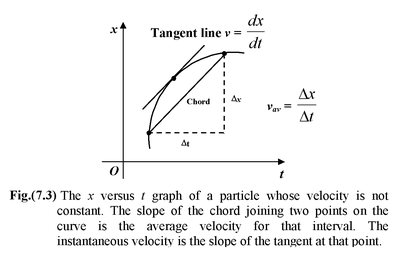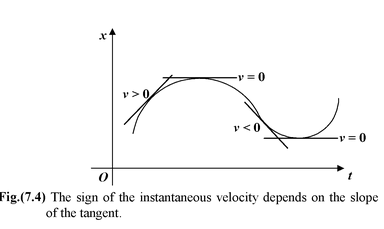### Average Acceleration

The average acceleration between two points in a time interval is equal to the slope of the chord connecting the points on a velocity versus time graph.

### Instantaneous Acceleration

The instantaneous acceleration at time t is the slope of the tangent drawn to the velocity versus time graph.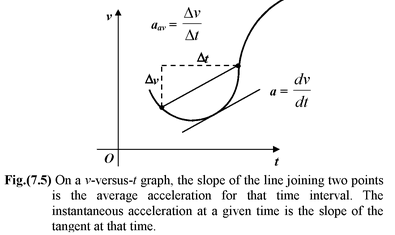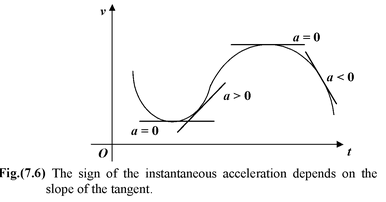### Displacement from Velocity Time Graphs

Given a velocity versus time graph, the displacement during an interval between time ti and tf is the area bounded by the velocity curve and the two vertical lines t = ti and t = tf, as shown in the Fig.(7.7 a).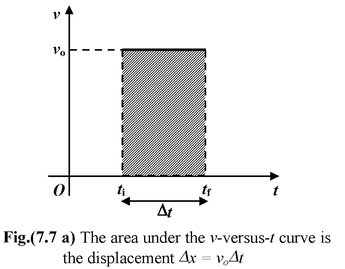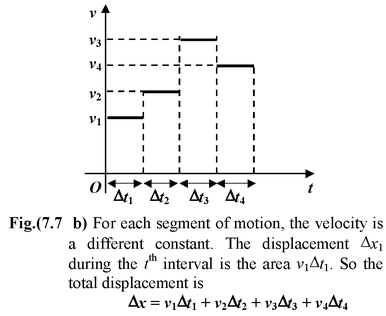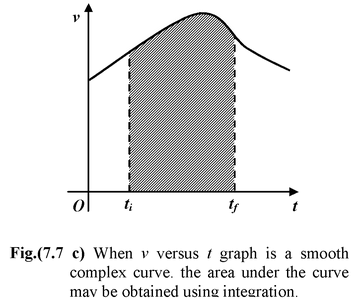### Velocity from Acceleration Time Graphs

Given an acceleration−versus−time graph, the change in velocity between t = ti and t = tf is the area bounded by the acceleration curve and the vertical lines t = ti and t = tf.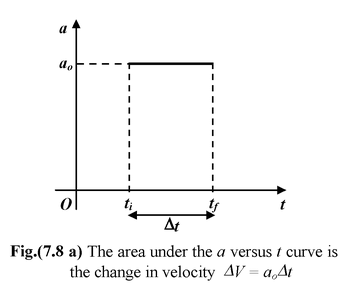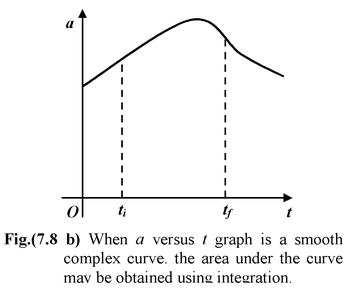Table 7.1 Variation of Displacement (x), velocity (v) and acceleration (a) with respect to time for different types of motion.

 Displacement Velocity Acceleration 1. At rest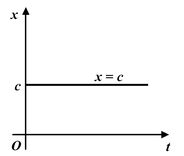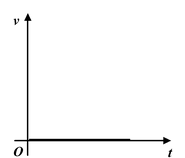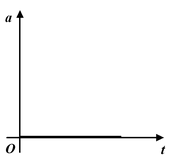2. Motion with constant velocity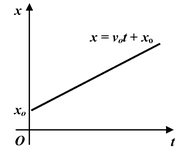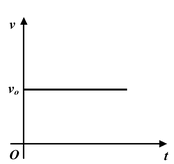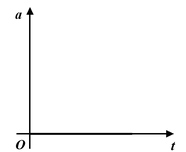3. Motion with constant acceleration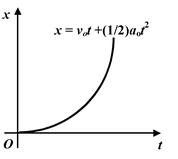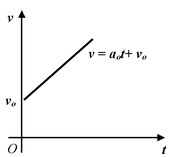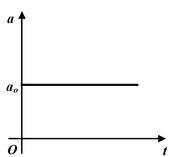4. Motion with constant deceleration.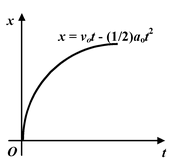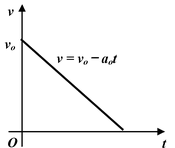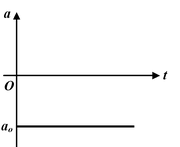Example: 7.4

 At t = 0 a particle is at rest at the origin. Its acceleration is 2 m/s2 for the first 2 second and −2 m/s2 for the next 2 s. Plot the x versus t and v versus t graphs. Solution It is given that x = 0 and v = 0 at t = 0. The acceleration versus time graph is plotted in Fig. (7.9a). The velocity at t = 2 s is equal to the sum of velocity at t = 0 and the area under the acceleration−time graph between t = 0 and t = 2 s.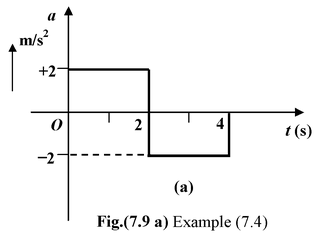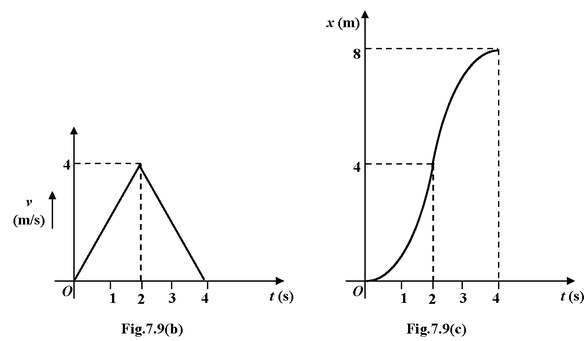v2 = v0 +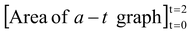Since at t = 0: velocity is zero, vo = 0

∴ v2 = 0 + (2) (2) = 4 m/s

Similarly velocity at t = 4s is

v4 = v0 +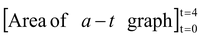= 0 +2 (+2) + 2 (−2) = 0

Note that area below the x−axis is taken as negative

The displacement at t = 2 s is

x2 = x0 +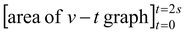∴ x2 = 0 + 1/2 (2) (4) = 4 m

and x4 = 0 + 1/2 (4) (4) = 8 m